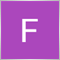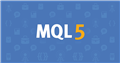# normalizedoubl19

No, not a programmer.. just trying to do a simple change.

Print("Point A:  " + NormalizeDouble(m_LastSwingPoints.swingValue, Digits() - 1));

Output:  0.9641999999999999

How can i get this down to 4 digits after the decimal?Documentation on MQL5: Conversion Functions / NormalizeDouble
• www.mql5.com
Calculated values of StopLoss, TakeProfit, and values of open prices for pending orders must be normalized with the accuracy, the value of which can be obtained by Digits(). Please note...23208

NormalizeDouble returns a double.

Floating-point has an infinite number of decimals, it's your not understanding floating-point and that some numbers can't be represented exactly. (like 1/10.)
Double-precision floating-point format - Wikipedia, the free encyclopedia

If you want to see the correct number of digits, convert it to a string with the correct/wanted accuracy.
question about decima of marketinfo() - MQL4 programming forum 2016.05.1819

William Roeder:
NormalizeDouble returns a double.

Floating-point has an infinite number of decimals, it's your not understanding floating-point and that some numbers can't be represented exactly. (like 1/10.)
Double-precision floating-point format - Wikipedia, the free encyclopedia

If you want to see the correct number of digits, convert it to a string with the correct/wanted accuracy.
question about decima of marketinfo() - MQL4 programming forum 2016.05.18

Ok thanks, so if I understanding you, I should be converting it to a string and using PrintFormat to do this?